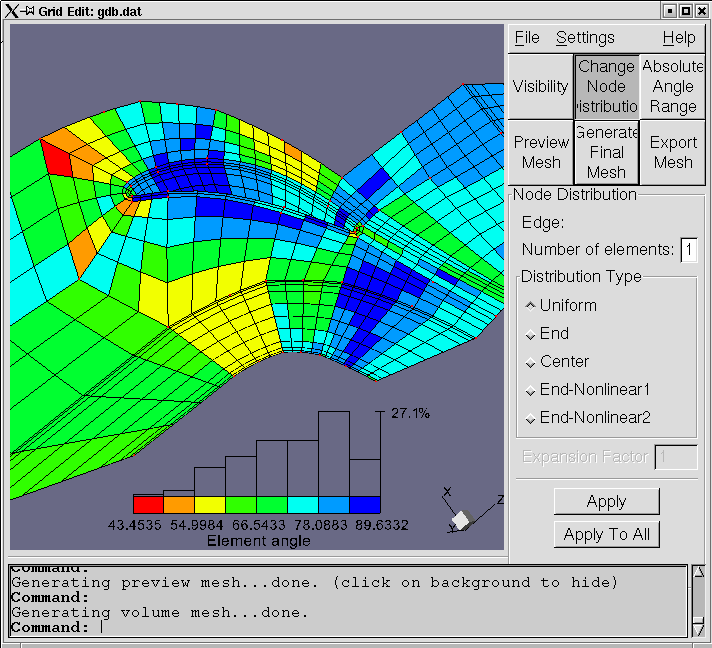## Investigations on water power turbines performed in LECAD

LECAD performs numerical simulations of Pelton , Francis and Kaplan turbines, as well as some derivatives such as Pipe and SAXO turbines. We investigate the flow properties, as well as the mechanicall stresses and eigenfrequencies of the turbine runners and other relevant parts like spiral cases by means of numerical simulations.

We perform two types of simulations:
- siumulation of stress-strain mchanical state of solid turbine parts under prescribed loads (pressure and other mechanical forces)
- estimation of turbine parameters by simulating the water flow through a water passage (flow rate, efficiency, transient phenomena)
The simulations are performed using numerical methods (Finite element method for the first type and Finite Volume Method for the last one). Complex geomtries are first modeled by a 3D Geometric modeler (like SDRC I-DEAS, ProEngineer, Unigraphics, ...). After that, the computational domains need to be discretized in order to transform the governing diferential equations into finite set of algebraic equations. The set of equations is then solved and finally the results (stress, velocity, pressure fields) are visualized or integral quantities (efficiency) computed.

Our main tools are EDS-SDRC-IDEAS (Geometric modeller and FE Analysis), AEA TASCflow (CFD pre- and postprocessor and solver) and ICCM Comet (CFD pre-postprocessor and solver with extended capabilities for simulating transient flow phenomena). We have also developed an unique tool for discretization of complex geometric domains, such as water turbine flow passage. It is based on "subdivision templates", which subdivide the whole comp. domain into smaller subdomains of simple (cuboidal for example) shapes. After that, the user interactively improves the location of subdomain vertices in order to generate mesh of highest possible quality. Currently, the modules have been coded, which generate multiblock structured all heksahedra grid, which can then be output to other commercial flow solver packages. See description of gridedit tool!The study of water turbine transient regimes (start of turbine, partial load rejection, emergency shut down) is the subject of current research. Have a look at the mpeg animation (13.3 MB)!
This document has been accessedtimes from 19. December 2001